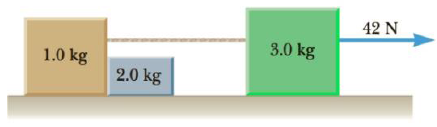Chapter 4, Problem 59P

Chapter
Section
Textbook Problem

Assume the three blocks portrayed in Figure P4.59 move on a frictionless surface and a 42-N force acts as shown on the 3.0-kg block. Determine (a) the acceleration given this system, (b) the tension in the cord connecting the 3.0-kg and the 1.0-kg blocks, and (c) the force exerted by the 1.0-kg block on the 2.0-kg block.Figure P4.59

(a)

To determine
The acceleration.

Explanation

Given Info: Masses of the blocks are 1 kg, 2 kg and 3 kg. The horizontal force applied is 42 N.

Formula for acceleration is,

a=Fm

• F is the horizontal force.
• m is the total mass.

Total mass is,

m=(1kg)+(2kg)+(3kg)=<

(b)

To determine
The tension on the cord.

(c)

To determine
The force exerted by the 1 kg block on the 2 kg block.

Still sussing out bartleby?

Check out a sample textbook solution.

See a sample solution

The Solution to Your Study Problems

Bartleby provides explanations to thousands of textbook problems written by our experts, many with advanced degrees!

Get Started

Find more solutions based on key concepts